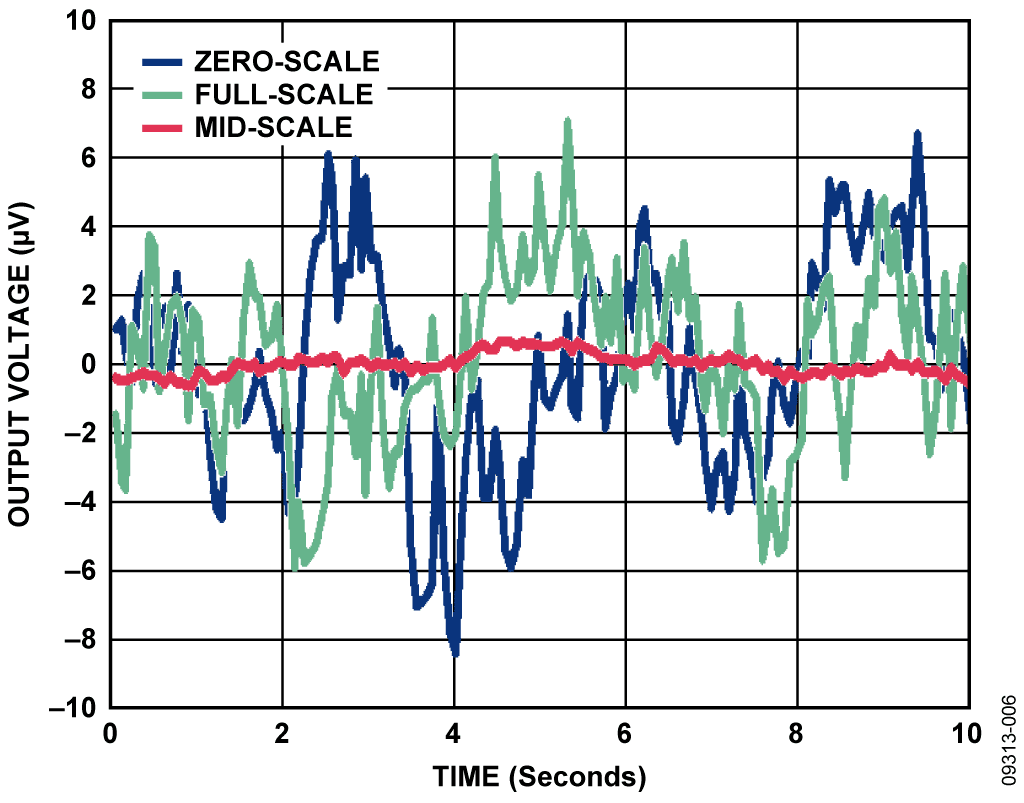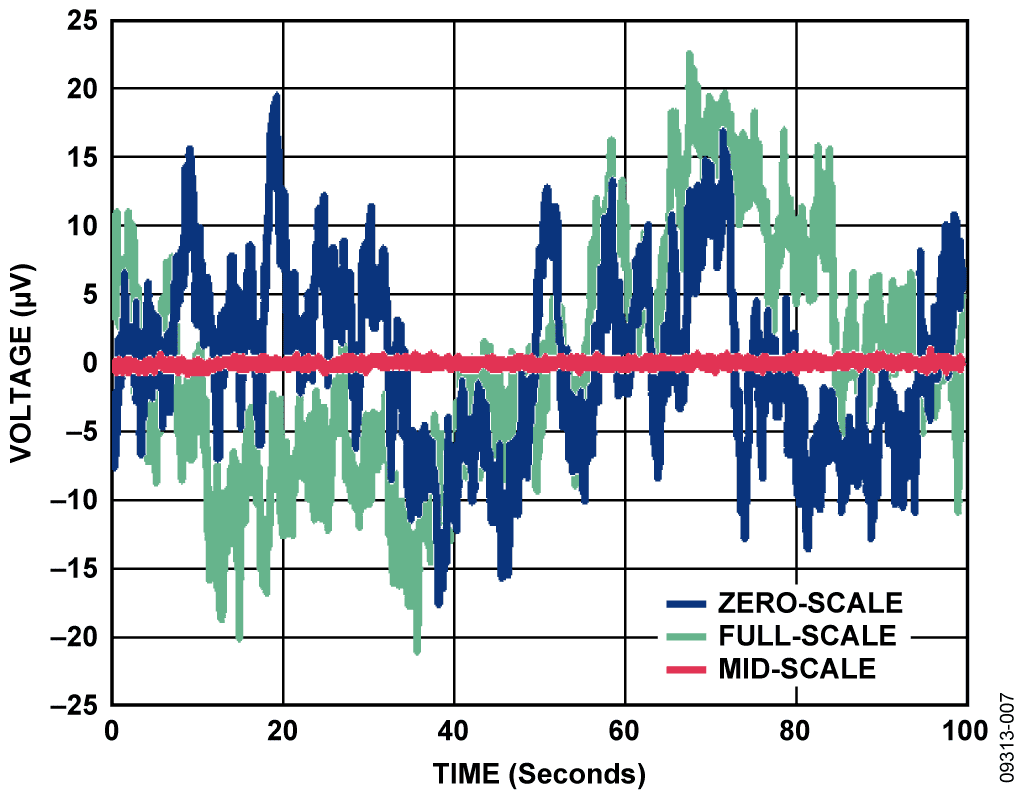## 設計リソース

### デバイス・ドライバ

コンポーネントのデジタル・インターフェースとを介して通信するために使用されるCコードやFPGAコードなどのソフトウェアです。

## 機能と利点

• 18-bit accurate voltage output source
• +/- 10V programmable output range
• Less than +/- 0.5 dB INL & DNL all codes

## 回路機能とその特長

The circuit shown in Figure 1 provides a programmable 18-bit voltage with an output range −10 V to +10 V, ±0.5 LSB integral nonlinearity, ±0.5 LSB differential nonlinearity, and low noise.

The digital input to the circuit is serial and is compatible with standard SPI, QSPI, MICROWIRE®, and DSP interface standards. For high accuracy applications, the circuit offers high precision, as well as low noise—this is ensured by the combination of the AD5781, ADR445 and AD8676 precision components.

The reference buffer is critical to the design because the input impedance at the DAC reference input is heavily code dependent and will lead to linearity errors if the DAC reference is not adequately buffered. With a high open-loop gain of 120 dB, the AD8676 has been proven and tested to meet the settling time, offset voltage, and low impedance drive capability required by this circuit application. The AD5781 is characterized and factory calibrated using the AD8676 dual op amp to buffer its voltage reference inputs, further enhancing confidence in partnering the components.

This combination of parts provides industry-leading 18-bit integral nonlinearity (INL) of ±0.5 LSB and differential nonlinearity (DNL) of ±0.5 LSB, with guaranteed monotonicity, as well as low power, small PCB area, and cost effectiveness.Figure 1. 18-bit Accurate, ±10 V Voltage Source (Simplified Schematic: All Connections and Decoupling Not Shown)

## 回路説明

The digital-to-analog converter (DAC) shown in Figure 1 is the AD5781, a high voltage, 18-bit converter with SPI interface, offering ±0.5 LSB INL, ±0.5 LSB DNL, and 7.5 nV/√Hz noise spectral density. The AD5781 also exhibits an extremely low temperature drift of 0.05 ppm/°C. The precision architecture of the AD5781 requires force-sense buffering of its voltage reference inputs to ensure specified linearity. The amplifiers (B1 and B2) chosen to buffer the reference inputs should have low noise, low temperature drift, and low input bias currents. The recommended amplifier for this function is the AD8676, an ultraprecision, 36 V, 2.8 nV/√Hz, dual op amp exhibiting low offset drift of 0.6 μV/°C and input bias currents of 2 nA. In addition, the AD5781 is characterized and factory calibrated using this dual op amp to buffer its voltage reference inputs, further enhancing confidence in partnering the components.

Figure 1 shows the AD5781 configured in a gain-of-two mode such that a single reference source can be used to generate a symmetrical bipolar output voltage range. This mode of operation uses an external op amp (A2), as well as on-chip resistors (see AD5781 data sheet) to provide the gain of two. These internal resistors are thermally matched to each other and to the DAC ladder resistance, resulting in ratiometric thermal tracking. The output buffer is again the AD8676, used for its low noise and low drift. This amplifier is also used (A1) to amplify the +5 V reference voltage from the low noise ADR445 to +10 V. R2 and R3 in this gain circuit are precision metal foil resistors with 0.01% tolerance and a temperature coefficient resistance of 0.6 ppm/°C. For optimum performance over temperature, R1 and R2 should be in a single package, such as the Vishay 300144 or VSR144 series. R2 and R3 are selected to be 1 kΩ to keep noise in the system low. R1 and C1 form a low-pass filter with a cutoff frequency of approximately 10 Hz. The purpose of this filter is to attenuate voltage reference noise.Figure 2. Integral Nonlinearity vs. DAC Code

### Linearity Measurements

The precision performance of the circuit shown in Figure 1 is demonstrated in the data in Figure 2 and Figure 3, which show integral nonlinearity and differential nonlinearity as a function of DAC code. As can be seen, both are significantly within the specifications of ±0.5 LSB and ±0.5 LSB, respectively.

The total unadjusted error for the circuit consists of the dc errors combined together—that is, INL error, offset error, and gain error. Figure 4 shows a plot of total unadjusted error as a function of DAC code. The maximum errors occur at DAC code zero and DAC code 262,143. This is expected, and due to the absolute error in the voltage reference output, the mismatch in external resistors R2 and R3 (see Figure 1), and the mismatch in the AD5781 internal resistors RFB and R1 (see Figure 5).Figure 3. Differential Nonlinearity vs DAC CodeFigure 5. Internal Gain-of-Two Circuitry (Simplified Schematic)

The specified voltage reference absolute error is 0.04%; the specified mismatch in resistors R2 and R3 in this case is 0.02%; the specified mismatch in internal resistors R1 and RFB is 0.01%. This results in a total gain error of 0.07% of full-scale range, or 184 LSBs. Figure 4 shows the measured value to be 20 LSBs, or 0.007% of full-scale range, indicating that all components are performing significantly better than their specified tolerances.

### Noise Measurements

To be able to realize high precision, the peak-to-peak noise at the circuit output must be maintained below 1 LSB, which is 76.29 μV for 18-bit resolution and a 20 V peak-to-peak voltage range. Figure 6 shows peak-to-peak noise measured in the 0.1 Hz to 10 Hz bandwidth over a period of 10 seconds. The peak-to-peak values for each of the three conditions are 1.34 μV for mid-scale output, 12.92 μV for full-scale output, and 15.02 μV for zero-scale output. Mid-scale output exhibits the lowest noise, as it represents the noise from the DAC core only. The noise contribution from each voltage reference path is attenuated by the DAC when mid-scale code is selected.Figure 6. Voltage Noise in 0.1 Hz to 10 Hz Bandwidth

A real application, however, will not have a high-pass cutoff at 0.1 Hz to attenuate 1/f noise, but will include frequencies down to dc in its pass band; therefore, the measured peak-to-peak noise will be more realistically shown in Figure 7. In this case, the noise at the output of the circuit was measured over a period of 100 seconds, effectively including frequencies as low as 0.01 Hz in the measurement. The upper frequency cutoff is at approximately 14 Hz and is limited by the measurement setup. For the three conditions shown in Figure 7, the peak-to-peak values are 1.61 μV for mid-scale output, 43.33 μV for full-scale output, and 36.89 μV for zero-scale output. The worst-case peak-to-peak value of 43.33 μV corresponds to approximately ½ LSB.Figure 7. Voltage Noise Measured Over 100 Second Period

As the time period over which the measurement is taken is increased, lower frequencies will be included, and the peak-to-peak value will increase. At low frequencies, temperature drift and thermocouple effects become contributors to noise. These effects can be minimized by choosing components with low thermal coefficients. In this circuit, the main contributor to low frequency 1/f noise is the voltage reference. It also exhibits the greatest temperature coefficient value in the circuit of 3 ppm/°C.

## バリエーション回路

The AD5781 will support a wide variety of output ranges from 0 V to +5 V up to ±10 V, and values in between. The gain-of-two configuration, as shown in Figure 1, can be used if a symmetrical output range is required. This mode is selected by setting the RBUF bit of the AD5781 internal control register to a Logic 0. If an asymmetrical range is required, individual references can be applied at VREFP and VREFN; and the output buffer should be configured for unity gain as described in the AD5781 data sheet. This is done by setting the RBUF bit of the AD5781 internal control register to a Logic 1.

## 回路の評価とテスト

The circuit shown in Figure 1 was constructed on a modified AD5781 evaluation board. Details of the AD5781 evaluation board and test methods can be found in Evaluation Board User Guide UG-184.